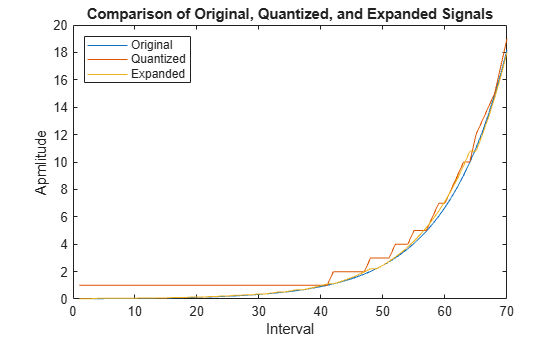# Quantize and Compand an Exponential Signal

When transmitting signals with a high dynamic range, quantization using equal length intervals can result in loss of precision and signal distortion. Companding is a operation that applies a logarithmic computation to compress the signal before quantization on the transmit side and applies an inverse operation to expand the signal to restore it to full scale on the receive side. Companding avoids signal distortion without the need to specify many quantization levels. Compare distortion when using 6-bit quantization on an exponential signal with and without companding. Plot the original exponential signal, the quantized signal and the expanded signal.

Create an exponential signal and calculate its maximum value.

```sig = exp(-4:0.1:4); V = max(sig);```

Quantize the signal by using equal-length intervals. Set partition and codebook values, assuming 6-bit quantization. Calculate the mean square distortion.

```partition = 0:2^6 - 1; codebook = 0:2^6; [~,qsig,distortion] = quantiz(sig,partition,codebook);```

Compress the signal by using the `compand` function configured to apply the mu-law method. Apply quantization and expand the quantized signal. Calculate the mean square distortion of the companded signal.

```mu = 255; % mu-law parameter csig_compressed = compand(sig,mu,V,'mu/compressor'); [~,quants] = quantiz(csig_compressed,partition,codebook); csig_expanded = compand(quants,mu,max(quants),'mu/expander'); distortion2 = sum((csig_expanded - sig).^2)/length(sig);```

Compare the mean square distortion for quantization versus combined companding and quantization. The distortion for the companded and quantized signal is an order of magnitude lower than the distortion of the quantized signal. Equal-length intervals are well suited to the logarithm of an exponential signal but not well suited to an exponential signal itself.

`[distortion, distortion2]`
```ans = 1×2 0.5348 0.0397 ```

Plot the original exponential signal, the quantized signal, and the expanded signal. Zoom in on axis to highlight the quantized signal error at lower signal levels.

```plot([sig' qsig' csig_expanded']); title('Comparison Between Original, Quantized, and Expanded Signals'); xlabel('Interval'); ylabel('Apmlitude'); legend('Original','Quantized','Expanded','location','nw'); axis([0 70 0 20])```